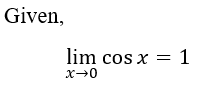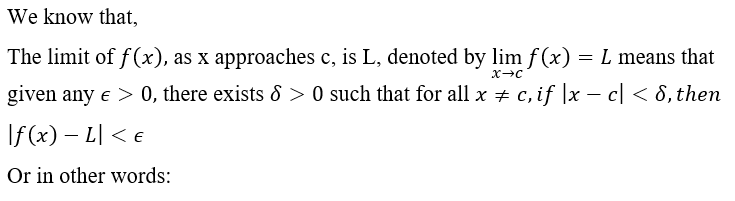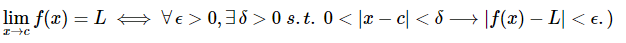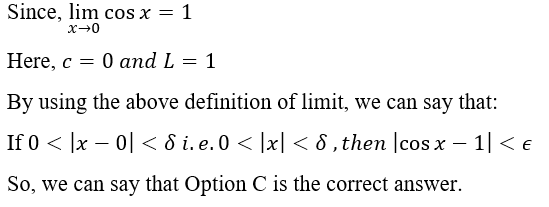# Given that limx→0cos x = 1, which of the following statements is true?(a) If |cos x − 1| is very small, then x is close to 0.(b) There is an E > 0 such that if if 0 < |cos x − 1| < E, then |x| < 10−5 .(c) There is a δ > 0 such that if 0 < |x| < δ, then |cos x − 1| < 10−5.(d) There is a δ > 0 such that if 0 < |x − 1| < δ, then |cos x| < 10−5.

Question
1 views

Given that lim
x→0
cos x = 1, which of the following statements is true?
(a) If |cos x − 1| is very small, then x is close to 0.
(b) There is an E > 0 such that if if 0 < |cos x − 1| < E, then |x| < 10−5 .
(c) There is a δ > 0 such that if 0 < |x| < δ, then |cos x − 1| < 10−5.
(d) There is a δ > 0 such that if 0 < |x − 1| < δ, then |cos x| < 10−5.

check_circle

Step 1Step 2Step 3...

### Want to see the full answer?

See Solution

#### Want to see this answer and more?

Solutions are written by subject experts who are available 24/7. Questions are typically answered within 1 hour.*

See Solution
*Response times may vary by subject and question.
Tagged in